# Function optimization with GeneticSharpIn this tutorial we will learn how to use GeneticSharp to optimize the inputs of math function and more about genetic algorithms meanwhile.

## Introduction

I created GeneticSharp a long time ago because three main reasons:

• I needed a fast, extensible, multi-platform and multithreading C# genetic algorithm library to use in my GA experiments;
• I wanted to study and understand GA better;
• and Genetic algorithm are pretty fun to implement and use!

As far as I know GeneticSharp has been used in a lot of different projects, since card games deck optimization, self managing distributed file system, context-sensitive code completion, even in airplanes trajectories optimization. These are pretty cool and exciting topics, but this tutorial is not about this advanced topics. Here I want to take a very simple sample and show how easy and fast you can add genetic algorithms on your project using GeneticSharp. So, let’s evolve!

## The problem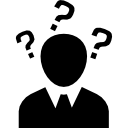In our sample we will optimize the input of a mathematical function, this function will be used as our fitness evaluation function. For this sample we will use a function that everyone saw in school time, the famous Euclidean distance or commonly know as distance of two points function: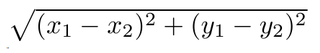We will consider our chromosome fitness as the result of this function. The higher the result, the better is the chromosome fitness.

Our chromosome will be the Euclidean distance function arguments, the X1, Y1, X2 and Y2.

## What our genetic algorithm is looking for?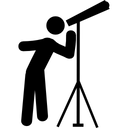The goal of our genetic algortim is find the input values of Euclidean distance function: X1, Y1 and X2, Y2 that result in the greatest distance in a rectangular area.

What? You can think: “this is a stupid goal”, because everyone knows that the longest distance between two points in a rectangular area are their diagonals. Yeah, you are right, but this is one of the best things about genetic algorithm, our GA code does not know anything about this, it just know that some X1, Y1 and X2, Y2 inputs generate a fitness value and greatest fitness are better. The other justification to use this so simple GA objective is that everyone can understand what is happening and we can focus to learn genetic algorithm.

We can easly create a brute force solution with four nested loops that will find the solution of our problem, but as I mencioned before this ‘problem’ is just for the tutorial purpose, because in normal applications genetic algoritm find solutions to problems that the solution is not so obvious or in some cases the solutions are even unknow.

## Creating the project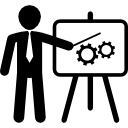Open your IDE (Visual Studio/Xamarin Studio) and create a new console project.

Install the GeneticSharp package:

``````install-package GeneticSharp
``````

## Using GeneticSharp

GeneticSharp implements all the classic components of a genetic algorithm, like gene, chromosome, population, fitness, selection, crossover, mutation, reinsetion and termination. When you use it to build your genetic algorithm code you just need to implement a few things, mostly you will need to code just your solution’s chromosome and fitness.

## Creating an Euclidean distance chromosome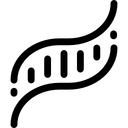Chromosome is the representation of a possible solution in genetic algoritms.

In GeneticSharp a chromosome needs to implement the IChromosome interface, but in most of cases you just need to inherit the ChromosomeBase class and override the methods GenerateGene and CreateNew.

Besides IChromosome and ChromosomeBase also exist the classes: BinaryChromosomeBase, FloatingPointChromosome and IntegerChromosome that are chromosome classes that can be directly used when your solution chromosome can be represented as numbers or a string representation of 0 and 1.

In our case we need to create a chromosome that represent the input variables of the Euclidean distance function: X1, Y1 and X2, Y2.

The FloatingPointChromosome is a perfect fit for this kind of representation, because it allows represent more than one number inside of it.

Open the Program.cs file and inside the Main function enter the code below:

In the code above we create two variables to represent our rectangle area size: maxWidth and maxHeight, then we created a new instance of FloatingPointChromome, this will be the template to all chromsome in our solution.

The constructor receive four arrays, they are:

1) The minimum values of numbers inside the chromosome. Our rectangle area will start at 0 points, so the min value of X1, Y1, X2 and Y2 are 0 (zero).

2) The maximum values. We use our previous created variables.

3) The total bits used to represent each number. The maximum value is 998, so 10 bits is what we need.

GeneticSharp will warn you if you try to use a total bits that cannot hold a number inside your floating point chromosome.

4) The number of fraction (scale or decimal) part of the number. In our case we will not use any.

## Creating the population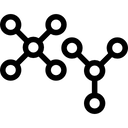The population represents the possible solutions to our problem, so we need to create a population of our Euclidean distance chromosome.

In GeneticSharp a population is represented by the IPopulation interface, but in most cases you can directly use the Population class.

We created a population that will have a minimum number of 50 chromosomes and a maximum number of 100 and used our chromosome template as the “Adam chromosome” (yeah, you get the reference) of our GA.

## Creating the fitness function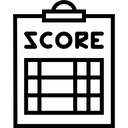The fitness function is where the genetic algoritm will evaluate and give a value (fitness) to each chromosome generated inside it. A good fitness function can guide your GA to a fast and optimum solution.

In GeneticSharp we represent a fitness function through the IFitness interface. Almost always you wil have to code a class that implement this interface, but for our tutorial we can use the simple and lower friction class FuncFitness. This class allow us to build our fitness evalution as its constructor argument.

We receive a IChromosome in the variable “c”, then we cast it to FloatingPointChromosme.

To allow us to evaluate the chromosome we need to convert it from its genotype (FloatingPointChromosome) to its phenotype (x1, y1 and x2, y2), we do this calling the ToFloatingPoints method. This method return an array of numbers using that configuration we used when we created our Euclidean distance chromosome. Now we have our X1, Y1 and X2 and Y2 numbers we just need to pass it to the Euclidean distance function and return the value as the fitness value of the current chromosome.

## Creating the selection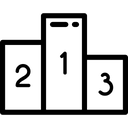A selection is the genetic algorithm operator responsible for decing which chromosomes of current population will be selected as parent of the next population.

You can code your our selection through the ISelection interface or extending the SelectionBase class.

Besides this, you can use the already implemented classic selections: Elite, Roulete Wheel, Stochastic Universal Sampling and Tournament.

Elite selection is a good option, because it will select the chromosomes with the best fitness (greatest distance). You can try the others selection options too and see how they change the GA speed and results.

## Creating the crossover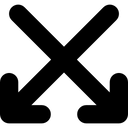The chromosomes selected by the selection need to cross to generate new possible solutions of the next generation of the GA. The crossover operator is responsible for crossing these selected chromosomes.

There are the ICrossover interface and CrossoverBase class if you want to code your crossover from scratch or you can use one of already available: Cut and Splice, Cycle (CX), One-Point (C1), Order-based (OX2), Ordered (OX1), Partially Mapped (PMX), Position-based (POS), Three parent, Two-Point (C2) and Uniform

Some of those classics crossovers, like OX1 and OX2, cannot be used in our tutorial because they need chromosomes with ordered genes and this is not the case of our chromosomes with 0101001100 genes. Do not worry, because GeneticSharp will warn you if you try to use an invalid chromosome in an ordered crossover

Uniform Crossover enables the parent chromosomes to contribute the gene level rather than the segment level, for example: if the mix probability is 0.5, the offspring has approximately half of the genes from first parent and the other half from second parent.

So, in our case Uniform crossover is a very good option, because using the 0.5f mix probability it will generate new chromosomes that are combination of X1, Y1 from one parent and X2, Y2 from another parent.

## Creating the mutation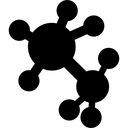The biology definition of mutations is: “In genetics, mutation may be small scale (affecting a gene) or large scale (involving a change in the chromosome). It may arise from faulty deletions, insertions, or exchanges of the genetic material. Such a change may result in the creation of a new character or trait.”

This biological process is one of the process responsible for I am able to write this tutorial and you be able read it. Without mutation, our species might have been found an local optima and perhaps we would never have evolved into what we are now.

The mutation operator has the same purpose in genetic algorithm, it avoid that our GA get stuck in optima local and end up never finding a better solution.

Like the other operators, you can create your own mutation implementing the IMutation interface or extending MutationBase or use some from the GeneticSharp menu: Flip-bit, Reverse Sequence (RSM), Twors and Uniform.

Flip-bit mutation is a mutation specific to chromosomes that implement IBinaryChromosome interface, as our FloatingPointChromosome does. It will randomly chose a gene and flip it bit, so a gene with value 0 will turn to 1 and vice-versa.

## Creating the termination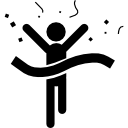A termination decide when a GA should be stopped. GeneticSharp use the generation number termination with just one generation as default termination. This mean that the genetic algoritm will run just one generation when you call the Start method, after this you can increment the expected generation number of the termination and call the method Resume how many times you want.

There are cases where you want to call the Start method in just wait until some condition be reached, this why termination exist.

If you have some special condition to terminate your GA you can implement the ITermination interface or extend the TerminationBase class, but for most of cases you just need to use some of the availables terminations: Generation number, Time evolving, Fitness stagnation, Fitness threshold, And e Or (allows combine others terminations).

In our tutorial we will use the fitness stagnation termination with a expected stagnant generations number of 100, this mean that if our GA generate the same best chromsome fitness in the last 100 generations then it will be terminated.

## Running the GA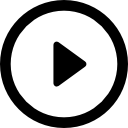Now that everything is set up, we just need to instantiate and start our genetic algorithm and watch it run.

So the GA ran, but where is the result? You can always get the best chromosome from the GeneticAlgorithm.BestChromosome property.

Another and better way to monitor the current best chromosome is use the GeneticAlgorithm.GenerationRan event. This event is raised right after a generation finish to run. Using this event you can see in realtime how the genetic algorithm is evolving.

Let’s replace our last “ga.Start();” line to the code below:

Now if your run the program you will see an output like that:

``````Generation  1: (178,330),(974,228) = 802.508566932466
Generation  2: (950,487),(45,520) = 905.601457596
Generation  3: (935,103),(38,617) = 1033.83025686038
Generation  4: (998,680),(49,65) = 1130.85189127489
Generation  8: (998,680),(57,1) = 1160.39734573981
Generation  9: (998,680),(49,1) = 1166.89416829462
Generation 11: (998,680),(17,19) = 1182.91250733095
Generation 12: (998,680),(25,3) = 1185.35142468384
Generation 14: (998,680),(17,3) = 1191.92701118819
Generation 16: (998,680),(17,1) = 1193.06412233375
Generation 17: (998,680),(16,1) = 1193.88651051932
Generation 23: (998,680),(0,1) = 1207.08119030991
Generation 32: (998,680),(0,0) = 1207.6439872744
``````

If we plot these generations the output image will be like this: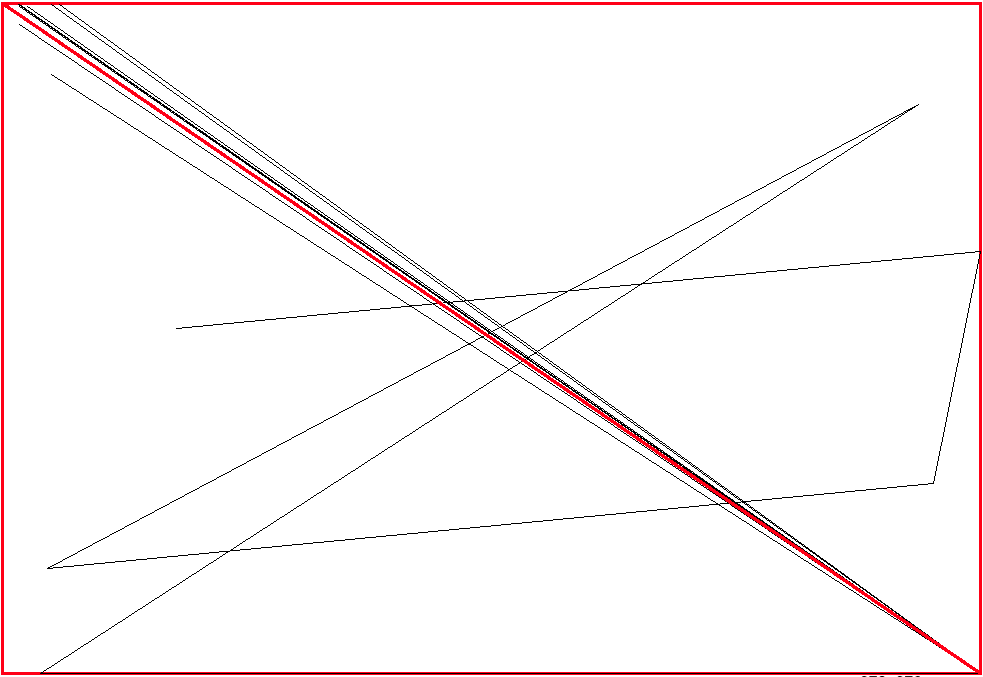We can see that in the first generations (black lines) the genetic algorithm as some intermediate results and at the end (red line) it found the best possible solution: a diagonal.

## Complete source code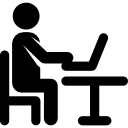When you finish the tutorial your source code will be this one:

## Conclusion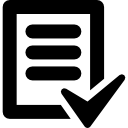In this tutorial we learn how genetic algoritm works and how to use GeneticSharp to solve a very simple sample. Now you can try it to solve more complex problems.

Let’s evolve!

Icons made by Freepik and Vignesh Oviyan from www.flaticon.com is licensed by CC 3.0 BY
Tutorials

News

Articles

Labs

See too